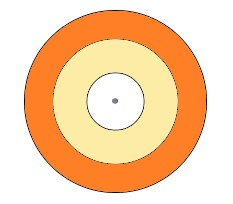"
">

# An archery target has three regions formed by three concentric circles as shown in the figure. If the diameters of the concentric circles are in the ratio 1:2:3, then find the ratio of the areas of three regions."

Given:

An archery target has three regions formed by three concentric circles as shown in the figure.

The diameters of the concentric circles are in the ratio 1:2:3.

To do:

We have to find the ratio of the areas of three regions.

Solution:

Let the diameters of the concentric circles be $k, 2k , 3k$.

This implies,

Radius of the concentric circles are $\frac{k}{2}, k$ and $\frac{3 k}{2}$.

Area of a circle of radius $r=\pi r^2$

Therefore,

Area of inner circle region $=\pi(\frac{k}{2})^{2}$

$=\frac{k^{2} \pi}{4}$

Area of middle region $=\pi(k)^{2}-\frac{k^{2} \pi}{4}$

$=\frac{4 k^{2} \pi-k^{2} \pi}{4}$

$=\frac{3 k^{2} \pi}{4}$

Area of the outer region $=\pi(\frac{3 k}{2})^{2}-\pi k^{2}$

$=\frac{9 \pi k^{2}}{4}-\pi k^{2}$

$=\frac{9 k^{2} \pi-4 k^{2} \pi}{4}$

$=\frac{5 \pi k^{2}}{4}$

The ratio of the three regions $=\frac{k^{2} \pi}{4}: \frac{3 k^{2} \pi}{4}: \frac{5 \pi k^{2}}{4}$

$=1: 3: 5$

The ratio of the areas of three regions is $1:3:5$.

Updated on: 10-Oct-2022

43 Views